### Home > MC2 > Chapter 5 > Lesson 5.2.4 > Problem5-82

5-82.
1. Find the perimeter and area of each triangle below. Homework Help ✎

1.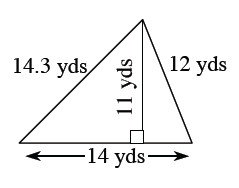2.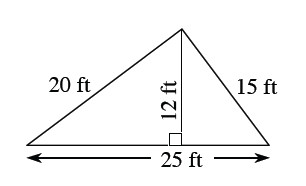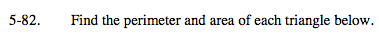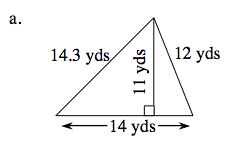The perimeter is the sum of all the sides of the shape.

14.3 + 12 + 14 = perimeter

Remember to include units.

The area of a triangle can be found using the formula:

$\text{Area} =\frac{1}{2}\text{(base)(height)}$

Remember to include units.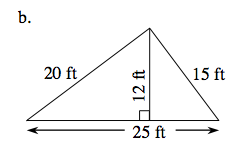See part (a).